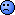## Questions and Ambiguities [BE/BA News]

Dear Helmut,

» Period ratios (second / first administration):
» Type 3: SAS according to R-doc - 2 outliers (#46: 3.524, #45: 26.08)
» Type 5: or is this SAS? - 2 outliers
» Type 6: Minitab, SPSS, Phoenix/WinNonlin - 2 outliers
» ...

SAS has 5 different percentile definitions. The default is:
Let n*p=j+g where j is the integer part, g is the fractional part, n is the number of values, x the ordered values.
Let y denote the percentile. Then (SAS PCTLDEF=5)
   y = 0.5*(xj+xj+1) if g=0   y = xj           if g>0
This corresponds to R's Type 2 I think.

» Don’t know what to do.Suggestions?

If the 'outlier' considerations based on the crippled EMA model makes any sense at all, which I'm not convinced at all, I would vote for an analysis of the residuals, not the period ratios also they appeared a natural choice on the first view.
Pro's:
• Period effects accounted for
• Analysis within the log domain (deemed necessary for AUC, Cmax)
• Distribution hopefully nearly normal, nearly symmetric because of the previous point, such that the 3xIQR rule can be applied reasonably
• Independent of order (first/second or second/first)
Con's:
• Period effects different from the whole model, because of throwing away all data under Test, residuals therefore may be biased
• Residuals not independent because of the model fitted (2 values of residual on a subject only different in sign, must throw away 1/2 of them)
But these Con's are unavoidable because of the EMA 'model', whatever here is modelled.
• simple residuals?
• studentized residuals?
• external studentized residuals (leave-one-out residuals)?
• other influence statistics (e.g. Cook's distance)?

Regards,

DetlewIng. Helmut Schütz